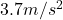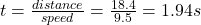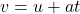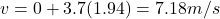## A particle travels horizontally between two parallel walls separated by 18.4 m. It moves toward the opposing wall at a constant rate of 9.5

Question

A particle travels horizontally between two parallel walls separated by 18.4 m. It moves toward the opposing wall at a constant rate of 9.5 m/s. Also, it has an acceleration in the direction parallel to the walls of 3.7 m/s 2 . 18.4 m 3.7 m/s 2 9.5 m/s What will be its speed when it hits the opposing wall? Answer in units of m/s

in progress 0
2 months 2021-07-22T18:30:15+00:00 1 Answers 1 views 0

7.18m/s

Explanation:

We are given that

Distance,s=18.4 m

Speed,v=9.5 m/s

Acceleration,a=We have to find the speed when it hits the opposing wall.

In perpendicular direction,a=0

Time,In parallel direction

Initial velocity,u=0Substitute the values